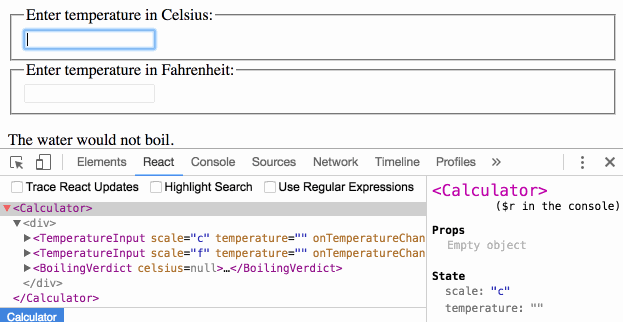# 状态提升

``````function BoilingVerdict(props) {
if (props.celsius >= 100) {
return <p>The water would boil.</p>;
}
return <p>The water would not boil.</p>;
}``````

``````class Calculator extends React.Component {
constructor(props) {
super(props);
this.handleChange = this.handleChange.bind(this);
this.state = {temperature: ''};
}

handleChange(e) {
this.setState({temperature: e.target.value});
}

render() {
const temperature = this.state.temperature;
return (
<fieldset>
<legend>Enter temperature in Celsius:</legend>
<input
value={temperature}
onChange={this.handleChange} />
<BoilingVerdict
celsius={parseFloat(temperature)} />
</fieldset>
);
}
}``````

## 添加第二个输入框

``````const scaleNames = {
c: 'Celsius',
f: 'Fahrenheit'
};

class TemperatureInput extends React.Component {
constructor(props) {
super(props);
this.handleChange = this.handleChange.bind(this);
this.state = {temperature: ''};
}

handleChange(e) {
this.setState({temperature: e.target.value});
}

render() {
const temperature = this.state.temperature;
const scale = this.props.scale;
return (
<fieldset>
<legend>Enter temperature in {scaleNames[scale]}:</legend>
<input value={temperature}
onChange={this.handleChange} />
</fieldset>
);
}
}``````

``````class Calculator extends React.Component {
render() {
return (
<div>
<TemperatureInput scale="c" />
<TemperatureInput scale="f" />
</div>
);
}
}``````

## 编写转换函数

``````function toCelsius(fahrenheit) {
return (fahrenheit - 32) * 5 / 9;
}

function toFahrenheit(celsius) {
return (celsius * 9 / 5) + 32;
}``````

``````function tryConvert(temperature, convert) {
const input = parseFloat(temperature);
if (Number.isNaN(input)) {
return '';
}
const output = convert(input);
const rounded = Math.round(output * 1000) / 1000;
return rounded.toString();
}``````

## 状态提升

``````class TemperatureInput extends React.Component {
constructor(props) {
super(props);
this.handleChange = this.handleChange.bind(this);
this.state = {temperature: ''};
}

handleChange(e) {
this.setState({temperature: e.target.value});
}

render() {
const temperature = this.state.temperature;
// ...  ``````

``````  render() {
// Before: const temperature = this.state.temperature;
const temperature = this.props.temperature;
// ...``````

``````  handleChange(e) {
// Before: this.setState({temperature: e.target.value});
this.props.onTemperatureChange(e.target.value);
// ...``````

`onTemperatureChange` 的 prop 和 `temperature` 的 prop 一样，均由父组件 `Calculator` 提供。它通过修改父组件自身的内部 state 来处理数据的变化，进而使用新的数值重新渲染两个输入框。我们将很快看到修改后的 `Calculator` 组件效果。

``````class TemperatureInput extends React.Component {
constructor(props) {
super(props);
this.handleChange = this.handleChange.bind(this);
}

handleChange(e) {
this.props.onTemperatureChange(e.target.value);
}

render() {
const temperature = this.props.temperature;
const scale = this.props.scale;
return (
<fieldset>
<legend>Enter temperature in {scaleNames[scale]}:</legend>
<input value={temperature}
onChange={this.handleChange} />
</fieldset>
);
}
}``````

``````{
temperature: '37',
scale: 'c'
}``````

``````{
temperature: '212',
scale: 'f'
}``````

``````class Calculator extends React.Component {
constructor(props) {
super(props);
this.handleCelsiusChange = this.handleCelsiusChange.bind(this);
this.handleFahrenheitChange = this.handleFahrenheitChange.bind(this);
this.state = {temperature: '', scale: 'c'};
}

handleCelsiusChange(temperature) {
this.setState({scale: 'c', temperature});
}

handleFahrenheitChange(temperature) {
this.setState({scale: 'f', temperature});
}

render() {
const scale = this.state.scale;
const temperature = this.state.temperature;
const celsius = scale === 'f' ? tryConvert(temperature, toCelsius) : temperature;
const fahrenheit = scale === 'c' ? tryConvert(temperature, toFahrenheit) : temperature;

return (
<div>
<TemperatureInput
scale="c"
temperature={celsius}
onTemperatureChange={this.handleCelsiusChange} />
<TemperatureInput
scale="f"
temperature={fahrenheit}
onTemperatureChange={this.handleFahrenheitChange} />
<BoilingVerdict
celsius={parseFloat(celsius)} />
</div>
);
}
}``````

• React 会调用 DOM 中 `<input>``onChange` 方法。在本实例中，它是 `TemperatureInput` 组件的 `handleChange` 方法。
• `TemperatureInput` 组件中的 `handleChange` 方法会调用 `this.props.onTemperatureChange()`，并传入新输入的值作为参数。其 props 诸如 `onTemperatureChange` 之类，均由父组件 `Calculator` 提供。
• 起初渲染时，用于摄氏度输入的子组件 `TemperatureInput``onTemperatureChange` 方法为 `Calculator` 组件中的 `handleCelsiusChange` 方法，而，用于华氏度输入的子组件 `TemperatureInput` 中的 `onTemperatureChange` 方法为 `Calculator` 组件中的 `handleFahrenheitChange` 方法。因此，无论哪个输入框被编辑都会调用 `Calculator` 组件中对应的方法。
• 在这些方法内部，`Calculator` 组件通过使用新的输入值与当前输入框对应的温度计量单位来调用 `this.setState()` 进而请求 React 重新渲染自己本身。
• React 调用 `Calculator` 组件的 `render` 方法得到组件的 UI 呈现。温度转换在这时进行，两个输入框中的数值通过当前输入温度和其计量单位来重新计算获得。
• React 使用 `Calculator` 组件提供的新 props 分别调用两个 `TemperatureInput` 子组件的 `render` 方法来获取子组件的 UI 呈现。
• React 调用 `BoilingVerdict` 组件的 `render` 方法，并将摄氏温度值以组件 props 方式传入。
• React DOM 根据输入值匹配水是否沸腾，并将结果更新至 DOM。我们刚刚编辑的输入框接收其当前值，另一个输入框内容更新为转换后的温度值。

## 学习小结